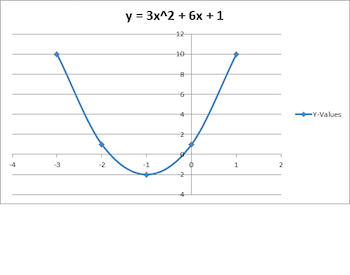# Vertex Form: Equation & Functions

An error occurred trying to load this video.

Try refreshing the page, or contact customer support.

Coming up next: Vertex: Definition & Function

### You're on a roll. Keep up the good work!

Replay
Your next lesson will play in 10 seconds
• 0:00 Vertex Form
• 0:33 Vertex of a Quadratic Function
• 1:49 Converting to and from…
• 3:38 From Vertex to Graph
• 5:28 Lesson Summary

Want to watch this again later?

Timeline
Autoplay
Autoplay
Speed

#### Recommended Lessons and Courses for You

Lesson Transcript
Instructor: Glenda Boozer
Just as y = mx + b is a useful format for graphing linear functions, y = a(x - h)^2 + k is a useful format for graphing quadratic functions. We will explore its uses and learn how to convert any quadratic equation into vertex form.

## Vertex Form

A quadratic equation can be written as ax2 + bx + c = 0, as long as a does not equal 0. We usually see this form, known as standard form, because it is used for more than one method of solving quadratic equations. In this lesson, however, we will look at vertex form, which is written as a(x - h)2 + k. We can make good use of this format for graphing quadratic functions.

## Vertex of a Quadratic Function

Let's graph the function associated with a quadratic equation: f'(x) = ax2 + bx + c or y = ax2 + bx + c.

Specifically, we can use y = 3x2 + 6x + 1 as an example.Notice that the point at (-1,-2) is the lowest point on the graph. This is what we call the vertex. We can also find the coordinates of the vertex from the equation itself, using this formula: x = -b/2a.

In this case, x = -6/2(3) = -6/6 = -1. Hey, it works! Now since we know that x is -1, we can determine that y = 3(-1)2 + 6(-1) + 1 = 3(1) + (-6) + 1 = 3 - 6 + 1 = -3 + 1 = -2. So, there is our (-1,-2). This means that we do not have to graph the equation to find the vertex.

## Converting to and from Vertex Form

Let's take the equation in standard form that we have already been working with:

y = 3x2 + 6x + 1

We already found the vertex to be (-1,-2).

The vertex form will look like:

a(x - h)2 + k

The a will be the same as in standard form, so we have:

y = 3(x - h)2 + k

Well, it turns out that the x-coordinate (our -1) is our h, and the y-coordinate (our -2) is the k. That gives us:

y = 3(x - (-1))2 + (-2), which is y = 3(x + 1)2 - 2

Thus, we use this method to convert from standard form to vertex form:

• Find the x-coordinate using the formula x = -b/2a
• Find the y-coordinate by evaluating f(x) = ax2 + bx + c with our value for x
• Use the a from the standard form, the x-coordinate for h and the y-coordinate for k in y = a(x - h)2 + k

If we want to go the other way, we can simply multiply (x - h) by itself, multiply that result by a, and add k.

For example:

3(x + 1)2 - 2 = 3(x2 + 2x + 1) - 2 = 3x2 + 6x + 3 - 2 = 3x2 + 6x + 1

That's what we started with, so we know how to convert standard form to vertex form or vertex form back to standard form.

To unlock this lesson you must be a Study.com Member.

### Register to view this lesson

Are you a student or a teacher?

### Unlock Your Education

#### See for yourself why 30 million people use Study.com

##### Become a Study.com member and start learning now.
Back
What teachers are saying about Study.com

### Earning College Credit

Did you know… We have over 160 college courses that prepare you to earn credit by exam that is accepted by over 1,500 colleges and universities. You can test out of the first two years of college and save thousands off your degree. Anyone can earn credit-by-exam regardless of age or education level.